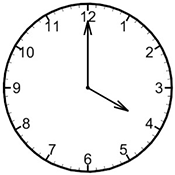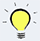# PHP Constants## What is a constant?

Constant is a name for a value and the name is used to access the value later in the script. If you have a value in your program that will not be changed during the script, then you can define it as constant. For example, the name of your site. Once defined, the value can’t be changed later or undefined. You can only change the constant where you it is defined.

## Defining a constant

Constant can be declared in 2 ways –

1. ### Using define() function-

Syntax
define(“CONSTANT_NAME”, value, [case_insensitive]);

Example

```<?php
define(“SITE_NAME”,”Schools of Web”);
?>
```

In the above example a constant named SITE_NAME is defined with the value Schools of Web.
The third parameter case_insesitive is optional. By default it is false, that means if you don’t mention the third parameter (as I did in the above example), the defined constant name will be case sensitive.

2. ### Using CONST keyword-

Syntax
const CONSTANT_NAME = value;

Example

```<?php
const SITE_NAME = ”Schools of Web”;
?>
```If you try to redefine a constant in the same script it will display nasty error on the screen. A nice way to define a constant is to verify whether it is already existed before defining it:

```<?php
const SITE_NAME = ”Schools of Web”;
if (!defined("SITE_NAME")) {  // checking whether the constant SITE_NAME is already defined/created.
define("SITE_NAME"," Schools of Web ");  // if not defined, then the constant is defined here.
}
?>```

## Constant naming rules

Constant naming rules are same as those of variable except constant name can’t be started with dollar (\$) sign. Here are the rules of valid constant names –

1. First character must be a letter or underscore. Ex. TODAY, _TODAY etc.
2. Other than the first character, there may have any number of letters (A-Z,a-z), numbers (0-9), or underscores(_). ex. _4TODAY etc.
3. Constant name is case-sensitive. That means TODAY and TODAy are 2 different constants unless case_insensitive are set as true in the third parameter in the define() function. (remember, false indicates constant name case insensitive)Though you’re free to use smaller letter, It is good practice to use only uppercase letter as constant name.

```<?php
const SITE_NAME = ”Schools of Web”;
?>
```

## Supporting data type

Constant can only contain scalar data type value. That is boolean, integer, float, and string.

```<?php
const IS_IT_YEAR_2002 = FALSE;  // boolean as a value stored in constant IS_IT_2002.
const DAYS_OF_WEEK = 7;  // number as a value stored in constant DAYS_OF_WEEK.
const REMAINING_MONEY = 100.25;  // float as a value stored in constant DAYS_OF_WEEK.
const SITE_NAME = “Schools of Web”;  // string as a value stored in constant SITE_NAME.
?>```

## Scope of a constant

1. The scope of a defined constant is global. The means after defining a constant, you can access constants anywhere in your script.
2. If another php script is included later after defining the constant, the constant can be accessed in that script too. (you’ll learn how to include a PHP script in another PHP script later.)

## Accessing value of a constant

You can access constant in 2 ways.

1. ### Using echo/print

To display the constant, use echo or print in front of it.
Syntax
echo CONSTANT_NAME;
print CONSTANT_NAME;

Example

```<?php
echo CONSTANT_NAME;
?>
```
2. ### Using constant() function

Syntax
echo constant(“CONSTANT_NAME”);

Example

```<?php
echo constant(“SITE_NAME”); // output: Schools of web
?>
```When constant is useful:
Sometimes you need to get the constant name dynamically – using variable name or return value from a function. In these cases, constant() function is useful. For example, in the following example the constant name (SITE_NAME) is being stored in a variable (\$any_variable), then the variable is using to get the constant name using constant() function.

```<?php
define("SITE_NAME", “Schools of Web”);
\$any_variable = "SITE_NAME";
echo constant(\$any_variable);  //Schools of Web
?>
```

## Difference between variable and constant

 Variable Constant Variable name must starts with \$ sign Constant name can’t be started with \$ sign Variable value can only be assigned by assignment operator (=) Constant may be not defined only with assignment operator (using cons keyword), but also with define() function. Variable can only be accessed within its scope. Once declared constant can be access anywhere in the script Variable’s value can be redefined after it is assigned. Constant can’t be redefined or undefined once it is set. Other than scalar value, variable can contain compound (array, object), special value (resource, NULL) On the other hand, constant can only contain scalar type (boolean, integer, float, and string) value. Variables used in heredoc syntax may be interpreted. Constant used in heredoc syntax are not interpreted.

## Predefined constants

PHP comes with lots of predefined constants. These constants are available to any scripts that PHP runs.

### Magic constants

1. There are eight predefined constants called magic constants.
2. These constants start and end with two underscores, and are case insensitive.
3. These are useful for beginners to learn.

List of magic constants with description-

 Constant name Description Version __LINE__ The line number of the script that contains this constant. __FILE__ Full path and file name where this constant is defined. If it is inside included file, it shows included file’s name. 4.0.2 __DIR__ The directory of the script that contains this constant. 5.3.0 __FUNCTION__ The name of the function that constants this constant. 4.3.0 __CLASS__ The name of the class that contains this constant. 4.3.0 __TRAIT__ The name of the trait that contains this constant. 5.4.0 __METHOD__ The name of the method that contains this constant. 5.0.0 __NAMESPACE__ The name of the current namespace (case sensitive) 5.3.0

Example

```<?php
echo __LINE__; // output: 2
echo __DIR__; //
?>
```

Explanation:
The above example shows the use of two constant. If you paste the above codes in your script, it will show 2 as output for line no 2, as the __LINE__ constant is written in the second line. The next constant __DIR__ will display the directory name of the script the script the line is written into.

 ‹‹ PHP Data Type : Previous Lesson Next Lesson: PHP Strings ››